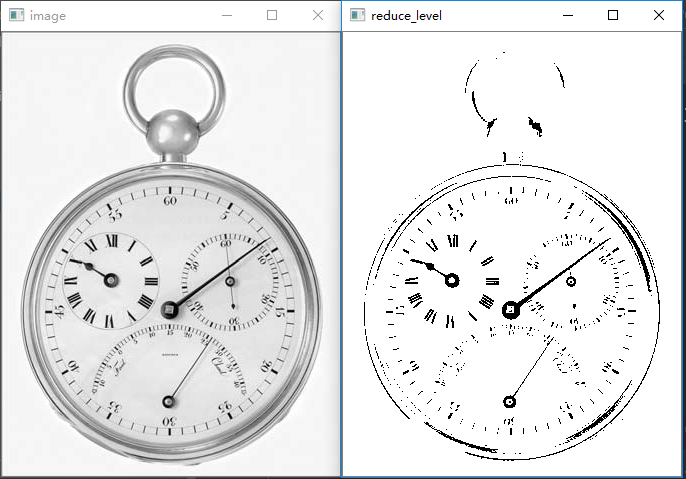2017-07-31 20:07:12 pianzang5201 阅读数 476
• ###### FFMpeg视频开发与应用基础——使用FFMpeg工具与SDK

FFMpeg是当今为的面向音视频开发的开源工程，广泛应用于多种音视频的客户端、播放器和流媒体服务器中。使用FFMpeg提供的工具和SDK，可以完成音视频的编码、解码、转码、封装、解封装、转封装、视频水印和视频缩放等多种需求的开发。

43973 人正在学习 去看看 殷汶杰

import cv2
import numpy as np

#第一种方法
#下面的None本应该是输出的图像尺寸，但是因为后面设置了缩放因子，所以这里设置了None
res = cv2.resize(img, None, fx = 2, fy = 2, interpolation = cv2.INTER_CUBIC)
#第二种方法
#直接设置输出图像的高宽值，所以没有设置缩放因子
height, width = img.shape[:2]
res = cv2.resize(img, (2*width, 2*height), interpolation = cv2.INTER_CUBIC)

while(1):
cv2.imshow('res', res)
cv2.imshow('img', img)

if cv2.waitKey(1)&0xFF==27:
break
cv2.destroyAllWindows()2018-01-14 01:14:54 u013165921 阅读数 3033
• ###### FFMpeg视频开发与应用基础——使用FFMpeg工具与SDK

FFMpeg是当今为的面向音视频开发的开源工程，广泛应用于多种音视频的客户端、播放器和流媒体服务器中。使用FFMpeg提供的工具和SDK，可以完成音视频的编码、解码、转码、封装、解封装、转封装、视频水印和视频缩放等多种需求的开发。

43973 人正在学习 去看看 殷汶杰

## 实验要求

(1.a) 编写一个以2 的幂次方将给定图像的灰度级数从256 减少到2 的程序。图像的灰度级数以参数变量的形式传递到所编写的程序中。

(1.b) 使用图2.21(a) 以(1.a)中编写的程序生成图2.21 所示的各个结果。

(2.a) 编写一个基于像素复制方式进行图像缩放的程序，假设缩放因子为整数。忽略混叠效应。

(2.b) 用编写的程序将图2.19 (a)从1024 x 1024 缩小到 256 x 256。

(2.c) 用编写的程序将(2.b)中的结果图像放大到1024 x 1024. 并解释与原图产生差异的原因。

(3.a) 编写一个以双线性插值技术进行图像缩放的程序，程序的输入参数为结果图像的水平和垂直方向的像素数。忽略混叠效应。

(3.b) 用编写的程序将图2.19 (a)从1024 x 1024 缩小到 256 x 256。

(3.c) 用编写的程序将(3.b)中的结果图像放大到1024 x 1024. 并解释与原图产生差异的原因。

## 技术论述

1、 图像灰度级变化
灰度图像是一种具有从黑到白256级灰度色阶或等级的单色图像。该图像中的每个像素用8位数据表示，因此像素点值介于黑白间的256种灰度中的一种。该图像只有灰度等级，而没有颜色的变化。
灰度即色阶或灰阶，又称中间色调，是指亮度的明暗程度。图像灰度级指图像中的色度分量亮度的最大值与最小值之差的级别，级数越多，图像亮度范围就越丰富，图像质量越好，反之，级数越少时图像质量越差。当图像只有2个灰度色阶时，称之为二值图像。
本实验中，将给定图像的灰度级数以2的幂次方从256减少到2，所采用量化方法为：

2、 像素复制方法
图像缩放中，像素复制方法的原理是对原来输入图像的整行或是整列像素进行简单的复制与删除，达到改变图像大小的目的。该方法计算量小、运算速度快，但容易产生失真，不适合处理具有大量细节的图像。

3、双线性插值技术
双线性插值又称双线性内插，当图像放大时，结果图像中新的像素值，是由原图像像素位置的临近点像素值通过加权平均计算得出的。
当对相邻四个像素点采用双线性插值时，所得表面在邻域处是吻合的，但斜率不吻合，并且双线性灰度插值的平滑作用可能使得图像的细节产生退化，这种现象在进行图像放大时尤其明显。
双线性插值的运算量比像素复制方法大，但是其处理结果更接近于原图像的细节。

## 实验结果## 实验程序

% 主函数

% 灰度级数降低
subplot(2,4,1);imshow(f0);title('Fig2.21(a)原图像');      % 显示原图像
for i = 1:7
subplot(2,4,i+1);
imshow(grayleveldec(f0,i));                          % for循环显示灰度级降低后的图像
title(sprintf("%d级灰度图像", 2^(8-i)));
end

% 像素复制
f_shrink = pixel_duplication(f,-4);                      % 图像缩小：像素复制
f_zoom = pixel_duplication(f_shrink ,4);                 % 图像放大：像素复制
figure;imshow(f);title('Fig2.19(a)原图像');
figure;imshow(f_shrink);title('像素复制缩小');
figure;imshow(f_zoom);title('像素复制放大');

% 双线性插值
f1_shrink = bilinear(f,256,256);                        % 图像缩小：双线性插值
f1_zoom = bilinear(f1_shrink,1024,1024);                % 图像放大：双线性插值
figure;imshow(f1_shrink);title('双线性插值缩小');
figure;imshow(f1_zoom);title('双线性插值放大');
function imt = grayleveldec(ima,factor)
%功能： 将原图像的灰度级按照2的foctor次幂减少

if factor < 0
factor = 0;
end
if factor > 8
factor = 8
end
dfact = uint8(power(2, factor));
imt = (ima / dfact) * dfact;                           % 取整量化
return;
function img_duplication = pixel_duplication(img_input,factor)
% 像素复制方法

[M,N] = size(img_input);
if factor == 0                                        % 图像不进行缩放
img_duplication  = img_input;
elseif factor > 0                                     % 图像放大
for i = 1 : M * factor
for j = 1 : N * factor
img_duplication(i,j) = img_input(ceil(i/factor),ceil(j/factor));
% 像素坐标下标从1开始而非0，故需要向上取整即使用ceil(x)函数
end
end
else                                                  % 图像缩小
for i = 1 : M / abs(factor)
for j = 1 : N / abs(factor)
img_duplication(i,j) = img_input(i*abs(factor),j*abs(factor));
end
end
end

end
function img_bilinear=bilinear(img_input,x_pixel,y_pixel)
% 双线性插值

[M,N]=size(img_input);                               % 图像的行数列数
img_input=double(img_input);                         % 转换为双精度类型
n = x_pixel/M;                                       % 缩放因子

% 为解决边界溢出问题，扩大原图像的四边边缘
img_temp= zeros(M+2,N+2);
img_temp(2:M+1,1) = img_input(:,1);                  % 左边,长度M
img_temp(2:M+1,N+2) = img_input(:,N);                % 右边,长度M
img_temp(2:M+1,2:N+1) = img_input(:,:);              % 中间,图像复制
img_temp(1,:) = img_temp(2,:);                       % 上边,长度N+2
img_temp(M+2,:) = img_temp(M+1,:);                   % 下边,长度N+2

M1 = round((M+2)*n);                                 % 计算加边后缩放的图像的行数
N1 = round((N+2)*n);                                 % 计算加边后缩放的图像的列数
img_res = zeros(M1,N1);

for i=round(n+1):round(x_pixel+n)
for j=round(n+1):round(y_pixel+n)
x=i/n;                                       % 缩放后的图像坐标在原图像处的位置
y=j/n;
u=x-floor(x);
v=y-floor(y);
img_res(i,j)=u*v*img_temp(x-u,y-v)+(1-u)*v*img_temp(x-u,y-v+1)+...
u*(1-v)*img_temp(x-u+1,y-v)+(1-v)*(1-u)*img_temp(x-u+1,y-v+1);
end
end
img_bilinear=img_res(n+1:x_pixel+n,n+1:y_pixel+n);
img_bilinear=uint8(img_bilinear);
end 

2017-11-08 00:20:58 qq_30666517 阅读数 1214
• ###### FFMpeg视频开发与应用基础——使用FFMpeg工具与SDK

FFMpeg是当今为的面向音视频开发的开源工程，广泛应用于多种音视频的客户端、播放器和流媒体服务器中。使用FFMpeg提供的工具和SDK，可以完成音视频的编码、解码、转码、封装、解封装、转封装、视频水印和视频缩放等多种需求的开发。

43973 人正在学习 去看看 殷汶杰

src代表原图像，dst代表需要缩放后的图像。w0,h0分别是原图像src的列数以及行数，w1,h1是dst的列数以及行数matlab代码如下:

% 实现最近邻内插
% 利用仿射变换的思想，采用后向映射法
% src = rgb2gray(src);
% 获取图像的宽和高，即图像的列数和行数
[h0, w0, z0] = size(src);
% 定义我们想放大的图像的尺寸
dst = zeros(800,800,3);
[h1, w1, z1] = size(dst);
% 分别得到x轴（即高）的缩放因子和y轴(宽)的缩放因子
fh = h0/h1;
fw = w0/w1;
% 进行差值处理
for i=1:800
for j=1:800
index_x=round(i*fh);
index_y=round(j*fw);
% 防止下标出现0和上标超过src的大小
if index_x==0
index_x=index_x+1;
end
if index_y==0
index_y=index_y+1;
end
if index_x>h0
index_x=index_x-1;
end
if index_y>w0
index_y=index_y-1;
end
dst(i,j,:) = src(index_x,index_y,:);
end
end
figure('name','source image')
imshow(src)
figure('name','target image')
% 在matlab中必须先转换一下数据类型，才可以正常显示
dst = im2uint8(mat2gray(dst));
imshow(dst)

2016-11-27 00:05:15 qq_24894159 阅读数 3688
• ###### FFMpeg视频开发与应用基础——使用FFMpeg工具与SDK

FFMpeg是当今为的面向音视频开发的开源工程，广泛应用于多种音视频的客户端、播放器和流媒体服务器中。使用FFMpeg提供的工具和SDK，可以完成音视频的编码、解码、转码、封装、解封装、转封装、视频水印和视频缩放等多种需求的开发。

43973 人正在学习 去看看 殷汶杰

(x,y,1)=(w,v,1)T(0)

T$T$是一个3×3$3×3$的矩阵
T=100010001(1)

http://www.cnblogs.com/qiengo/archive/2012/06/30/2570874.html

## 我们以尺度变化为例

- 前向映射

- 反响映射

function [Image] = scale (img, scal)
T = [1,0,0;0,1,0;0,0,1];
tT = T.*scal;
tT(3,3) = 1;
flag = sign(scal);
[x,y,z] = size(img);
tx = ceil(x*scal);
%ceil为向上取整函数
ty = ceil(y*scal);
Image = zeros(tx,ty);
for i=1:tx
for j=1:ty
temp = ceil((tT^-1)*[i;j;1]);
Image(i,j) = img(temp(1),temp(2));
end;
end;

endfunction2019-11-19 12:49:06 qq_29598161 阅读数 20
• ###### FFMpeg视频开发与应用基础——使用FFMpeg工具与SDK

FFMpeg是当今为的面向音视频开发的开源工程，广泛应用于多种音视频的客户端、播放器和流媒体服务器中。使用FFMpeg提供的工具和SDK，可以完成音视频的编码、解码、转码、封装、解封装、转封装、视频水印和视频缩放等多种需求的开发。

43973 人正在学习 去看看 殷汶杰

## 打开、显示、存储图片

image = cv2.imread("images/2_20_a.jpg", cv2.IMREAD_UNCHANGED)
cv2.imwrite("images/full.jpg", image, [int(cv2.IMWRITE_JPEG_QUALITY), 100])
cv2.imshow("image", image)
cv2.waitKey(0)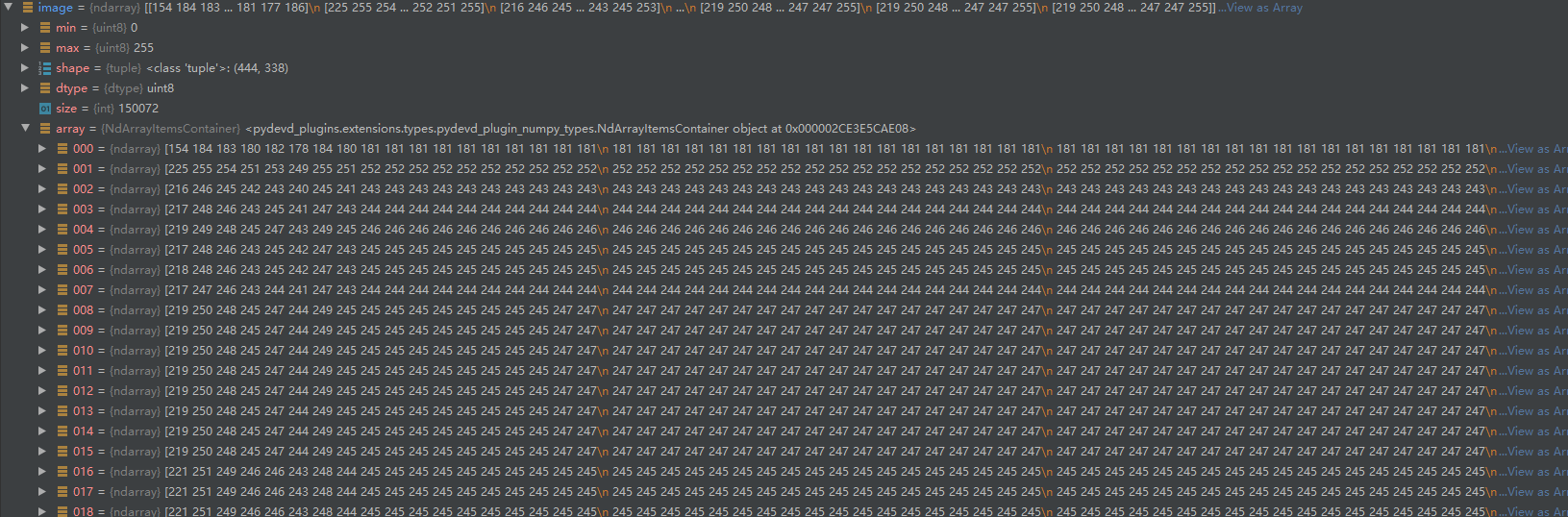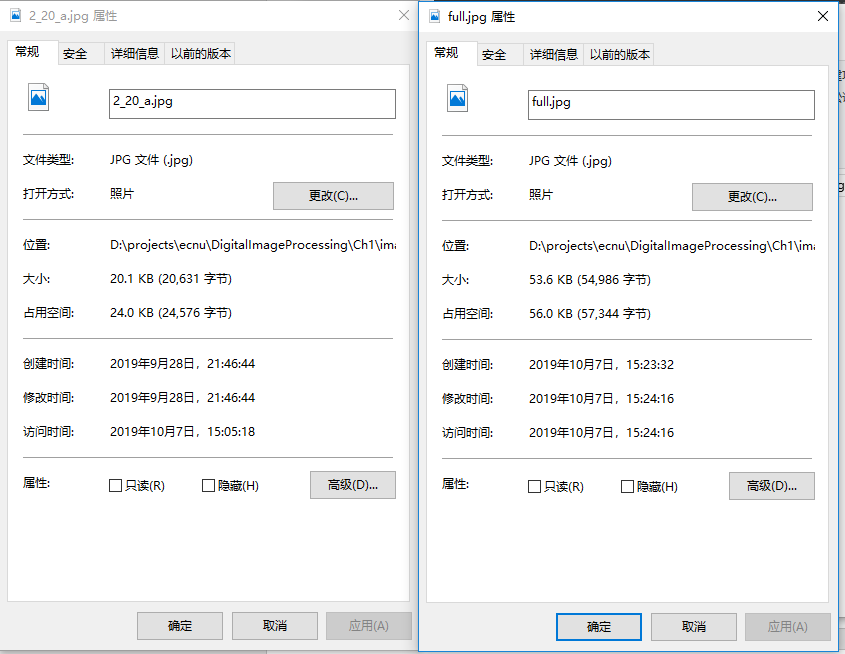## 图像缩放

### 最近领域插值

$h$, $w$, $h_0$, $w_0$ 分别为原图高、宽，和目标高、宽
$g(x, y)$为新图像x行y列的灰度等级，$f(x, y)$为原图像
$g(x, y) = f(\frac{xh}{h_0}, \frac{yw}{w_0}) (1)$

$g(\frac{xh_0}{h}, \frac{yw_0}{w}) = f(x, y) (2)$

Python关键代码：

def nearest(img, size):
"""
Nearest neighbor interpolation
:param img: source image
:param size: (height, width)
:return: destination image
"""
re = np.zeros([size, size, 1], np.uint8)
for x in range(size):
for y in range(size):
new_x = int(x * (img.shape / size))
new_y = int(y * (img.shape / size))
re[x, y] = img[new_x, new_y]
return re


### 双线性插值

$g(x, y) = f(\frac{xh}{h_0}, \frac{yw}{w_0}) = f(i+u, j+v) = (1-u)(1-v)f(i,j) + (1-u)vf(i,j+1) + u(1-v)f(i+1,j) + uvf(i+1,j+1)$

Python关键代码：

def bilinear(img, size):
"""
Bilinear interpolation
:param img: source image
:param size: (height, width)
:return: destination image
"""
re = np.zeros([size, size, 1], np.uint8)
for x in range(size):
for y in range(size):
new_x = x * (img.shape / size)
new_y = y * (img.shape / size)
i = int(new_x)
j = int(new_y)
u = new_x - i
v = new_y - j
if i + 1 >= img.shape:
i = img.shape - 2
if j + 1 >= img.shape:
j = img.shape - 2
# f(i+u,j+v)=(1−u)(1−v)f(i,j)+(1−u)vf(i,j+1)+u(1−v)f(i+1,j)+uvf(i+1,j+1)
re[x, y] =  (1-u)*(1-v)*img[i, j]
+ (1-u)*v*img[i, j+1]
+  u*(1-v)*img[i+1, j]
+  u*v*img[i+1, j+1]
return re


### 结果：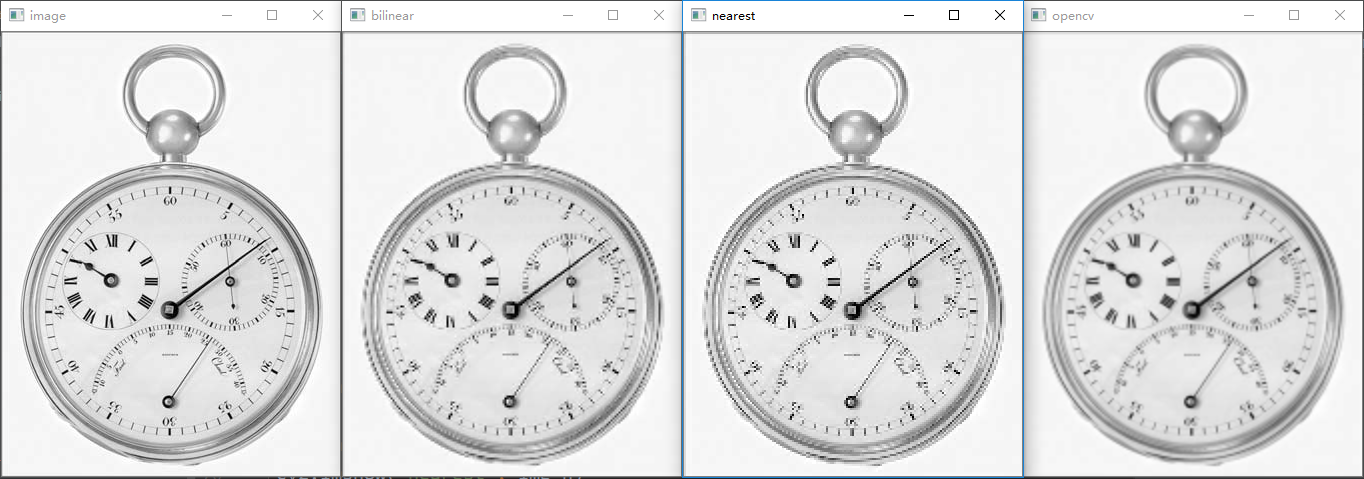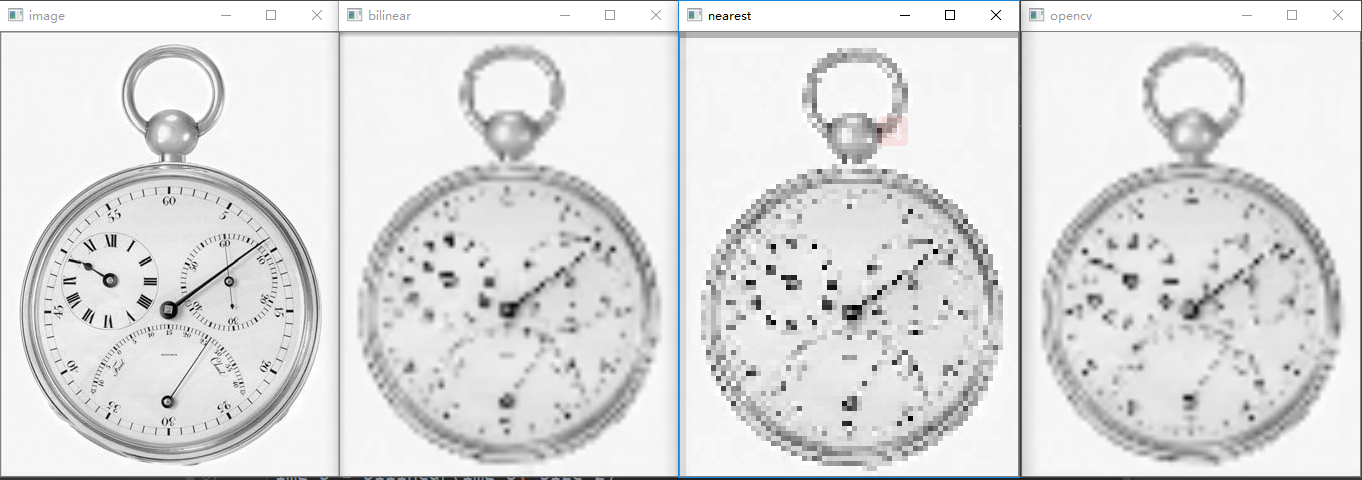OpenCV的效过较好，双线性插值其次，最近领域插值效果非常差。

### 降低灰度分辨率

def reduce_intensity_levels(img, level):
img = cv2.copyTo(img, None)
for x in range(img.shape):
for y in range(img.shape):
si = img[x, y]
ni = int(level * si / 255 + 0.5) * (255 / level)
img[x, y] = ni
return img


8灰度级图像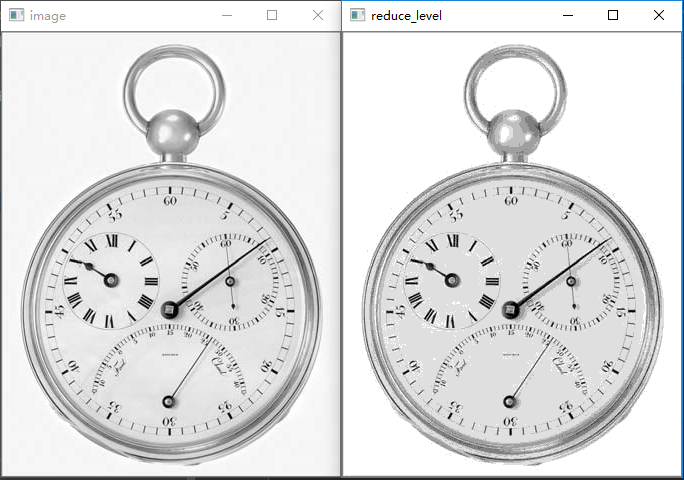4灰度级图像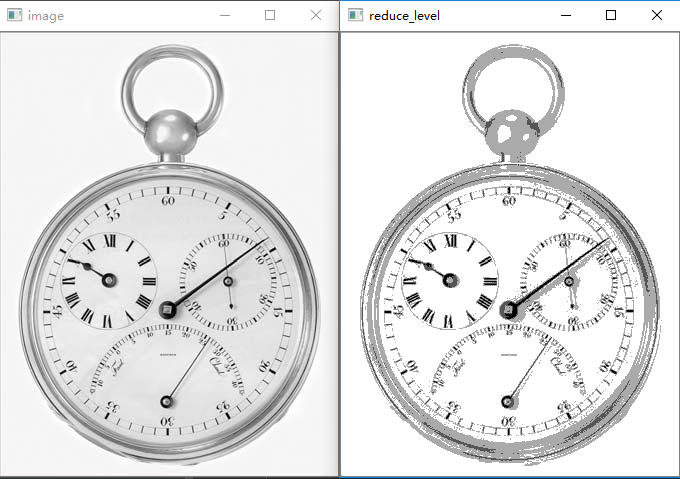2灰度级图像（2值化 0~127置0 128~256置255）